Last Updated On

Previously, Ms. Lim introduced a method to tackle open-ended questions on Heat Energy when it is tested together with Water Cycle.

Today, I will focus on the thought process behind answering questions testing on the melting and boiling points of substances. The questions commonly asked in the MCQ section require students to analyse the state of the substances at different temperatures, which tends to confuse them.

## Question Type #1

This question type typically provides information on the properties of the substance at a certain temperature. This allows students to identify if the substance is a solid, liquid or gas. Subsequently, students are expected to determine the possible melting and boiling points of that substance using the gathered information.

Let us have a look at the question below.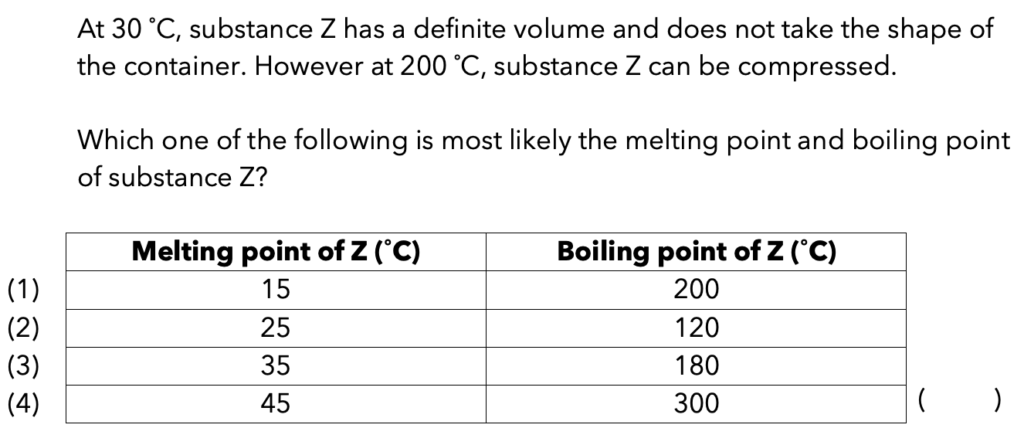Based on the information given about Substance Z in the question:

At 30˚C

• Definite volume
• Does not take the shape of the container

This suggests Substance Z is in a solid state.

200˚C

• Can be compressed

This suggests Substance Z is in a gaseous state.

We can represent these findings in the table below: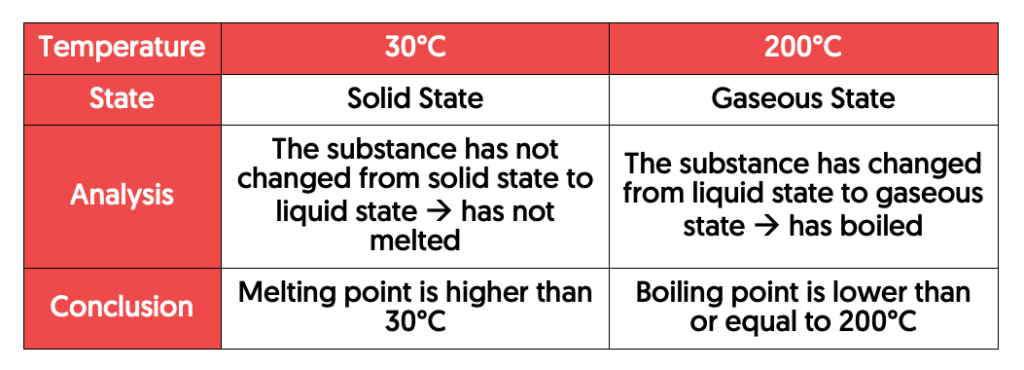With this information, we can safely conclude that the melting point of the substance is higher than 30˚C and the boiling point of the substance is lower than or equal to 200˚C.

Thus, the most suitable answer for this question would be Option 3.

## Question Type #2

This question type provides students with a list of substances and their respective melting and boiling points.

Students are to analyse the states of the substances at different temperatures. They may also be required to determine the temperature at which the substances will share the same state.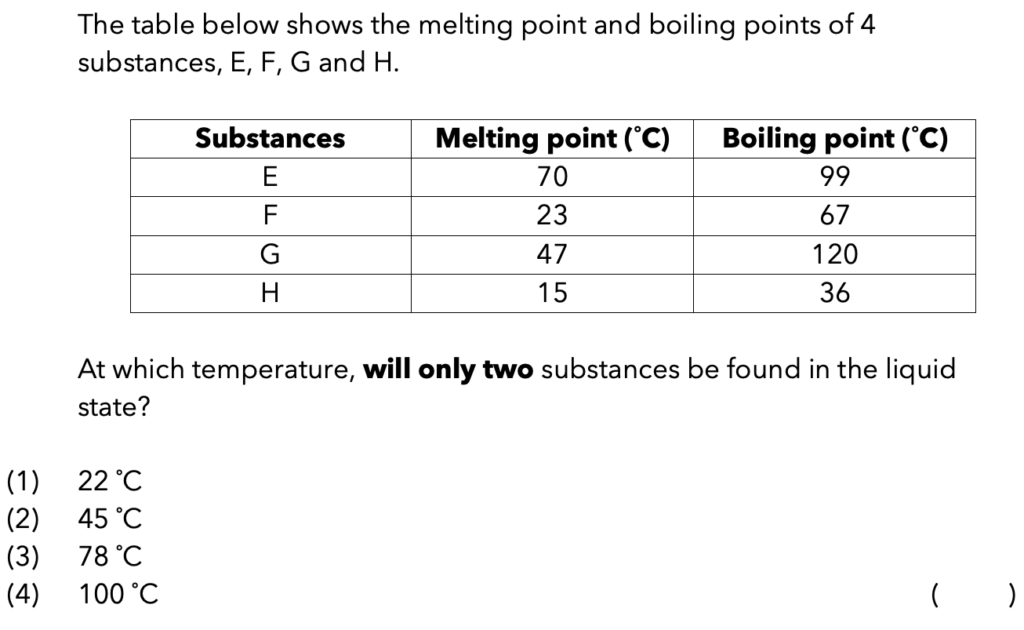I find it easier to tackle the question by first labelling “Solid (S)”, “Liquid (L)” and “Gas (G)” on the table.

Now, how do we know where to label Solid (S), Liquid (L) and Gas (G) on the table?

Substances at temperatures below the melting point have not melted yet and will be in the solid state. Thus, we write “Solid (S)before the melting point.

On the other hand, substances at temperatures between the melting point and the boiling point would have melted but have not boiled yet and will be in the liquid state. Thus, we write “Liquid (L)between the melting point and the boiling point.

Following that, substances at temperatures above the boiling point would have boiled and will be in the gaseous state. Thus, we write “Gas (G) after the boiling point.

In a nutshell, the following table shows how we should label “Solid (S)”, “Liquid (L)” and “Gas (G)” respectively.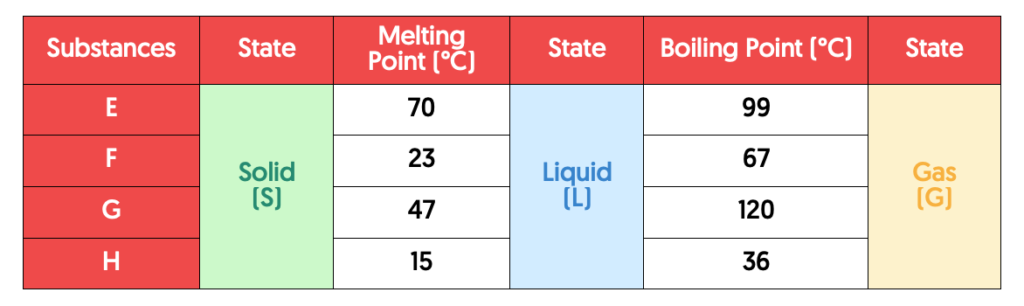Next, we will indicate the temperature given in each option into the respective positions in the table to determine the state of matter for each substance.

### Option (2)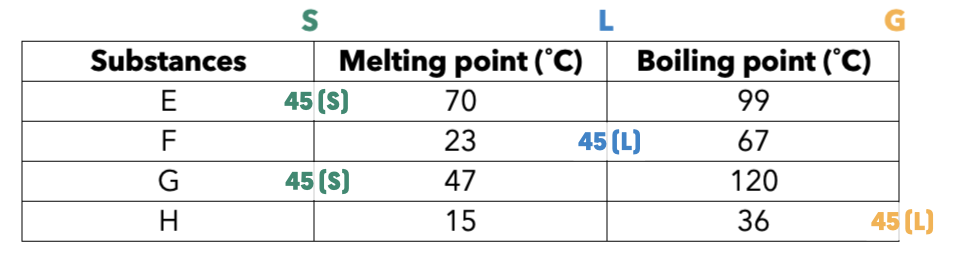### Option (3)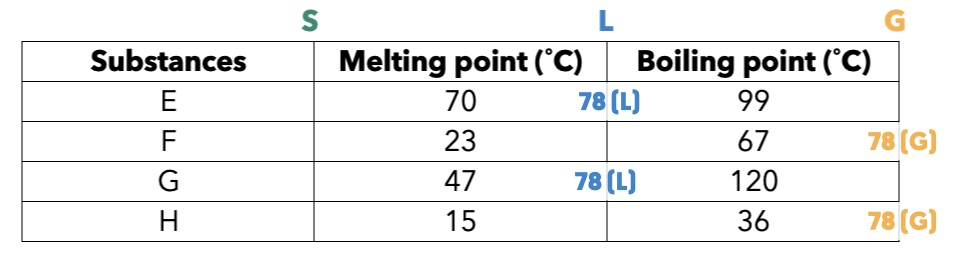### Option (4)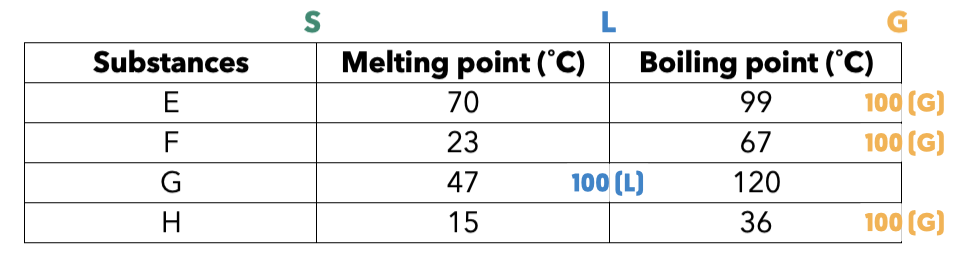Based on the options given and the 4 tables above, we can conclude that there will be two substances found in the liquid state only at Option (3): 78°C.

Thus, the correct answer is Option 3.

## Conclusion

We hope that you’ve gained a better understanding in tackling the different questions types testing on the melting and boiling points of substances.

• Heat is transferred from a hotter region (region of higher temperature) to a colder region (region of lower temperature).
• For a substance in the liquid state to freeze to the solid state, the liquid must first lose heat to reach its freezing point, then continue to lose heat until the process of freezing is complete.

Stay tuned for more articles on how to tackle Science questions!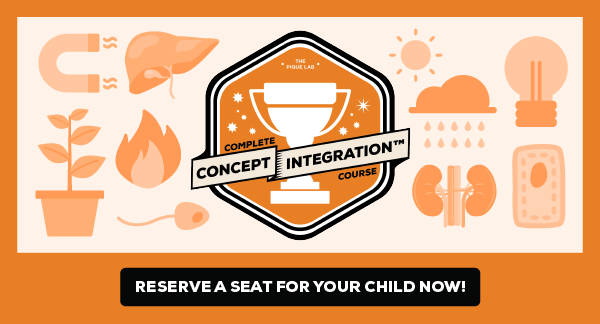If you like our methodology, we've some upcoming workshops: